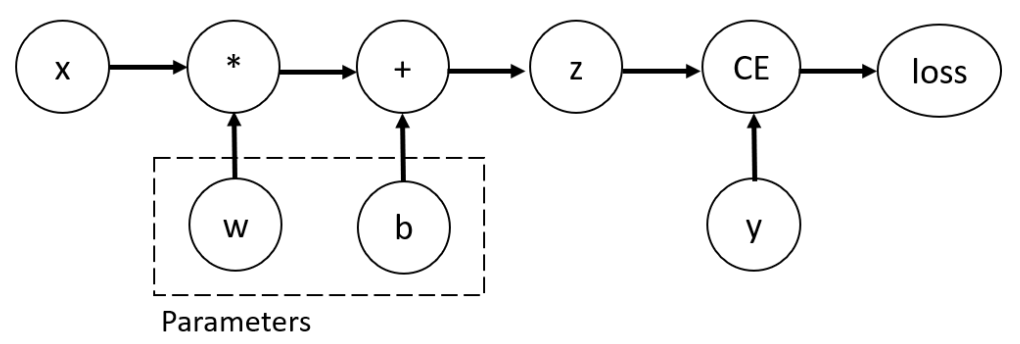# 自动微分运算-TORCH.AUTOGRAD

import torch

x = torch.ones(5)  # input tensor
y = torch.zeros(3)  # expected output
z = torch.matmul(x, w)+b
loss = torch.nn.functional.binary_cross_entropy_with_logits(z, y)


## 张量、函数和计算图print(f"Gradient function for z = {z.grad_fn}")


Gradient function for z = <AddBackward0 object at 0x7f9615a14580>
Gradient function for loss = <BinaryCrossEntropyWithLogitsBackward0 object at 0x7f9615a14bb0>


## 计算梯度

loss.backward()


tensor([[0.3313, 0.0626, 0.2530],
[0.3313, 0.0626, 0.2530],
[0.3313, 0.0626, 0.2530],
[0.3313, 0.0626, 0.2530],
[0.3313, 0.0626, 0.2530]])
tensor([0.3313, 0.0626, 0.2530])


• 我们只能从计算图中将 require_grad 设置为 True 的叶子结点获取 grad 属性。对于计算图中的其他节点，梯度不可获取。
• 在给定的计算图中，出于性能原因我们只能用 backward 进行一次梯度计算。如果我们想要对同一张计算图做几次 backward 调用，我们需要在 backward 调用时传递 retain_graph=True 参数。

## 禁用梯度追踪

z = torch.matmul(x, w)+b

z = torch.matmul(x, w)+b


True
False


z = torch.matmul(x, w)+b
z_det = z.detach()


False


• 为了把你神经网络中的某些参数标记为冻结参数(frozen parameters)
• 为了在你只做前向传递的时候加快计算速度，因为在不追踪梯度的张量上进行的运算会更加高效。

## 计算图的更多内容

• 运行指定的操作来计算、生成一个张量
• 维持这次运算在有向无环图中的梯度函数

• 从每个 .grad_fn 中计算梯度
• 在对应张量的 .grad 属性中累计它们
• 应用链式法则，一路传播到叶子张量。

## 选读: 张量梯度和 Jacobian （译为：雅各布）乘积

J =

$\begin{matrix} \frac{\partial y_1}{\partial x1} & ... & \frac{\partial y_1}{\partial x_n} \\ ... & ... & .. \\ \frac{\partial y_m}{\partial x1} & ... & \frac{\partial y_m}{\partial x_n} \end{matrix}\tag{1}$

PyTorch 允许你对一个给定的输入向量 $$v = < v_1,...,v_m >$$ 计算 Jacobian 乘积 $$v^T \cdot J$$。这可以通过把 $$v$$ 作为调用 backward 时的参数来实现的。$$v$$ 的大小应该和我们想要计算乘积的原始张量一致：

inp = torch.eye(4, 5, requires_grad=True)
out = (inp+1).pow(2).t()
out.backward(torch.ones_like(out), retain_graph=True)
out.backward(torch.ones_like(out), retain_graph=True)
out.backward(torch.ones_like(out), retain_graph=True)


First call
tensor([[4., 2., 2., 2., 2.],
[2., 4., 2., 2., 2.],
[2., 2., 4., 2., 2.],
[2., 2., 2., 4., 2.]])

Second call
tensor([[8., 4., 4., 4., 4.],
[4., 8., 4., 4., 4.],
[4., 4., 8., 4., 4.],
[4., 4., 4., 8., 4.]])